poseplot

Description

example

poseplot plots the pose (position and orientation) at the coordinate origin position with zero rotation. The default navigation frame is the north-east-down (NED) frame.

example

poseplot(quat) plots the pose with orientation specified by a quaternion quat. The position by default is [0 0 0].

example

poseplot(R) plots the pose with orientation specified by a rotation matrix R. The position by default is [0 0 0].

example

poseplot(___,position) specifies the position of the pose plot.

poseplot(___,frame) specifies the navigation frame of the pose plot.

example

poseplot(___,Name=Value) specifies pose patch properties using one or more name-value arguments. For example, poseplot(PatchFaceColor="r") plots the pose with red face color. For a list of properties, see PosePatch Properties.

poseplot(ax,___) specifies the parent axes of the pose plot.

example

p = poseplot(___) returns the PosePatch object. Use p to modify properties of the pose patch after creation. For a list of properties, see PosePatch Properties.

Examples

collapse all

Plot the default pose using the poseplot function with default settings.

poseplot
xlabel("North-x (m)")
ylabel("East-y (m)")
zlabel("Down-z (m)");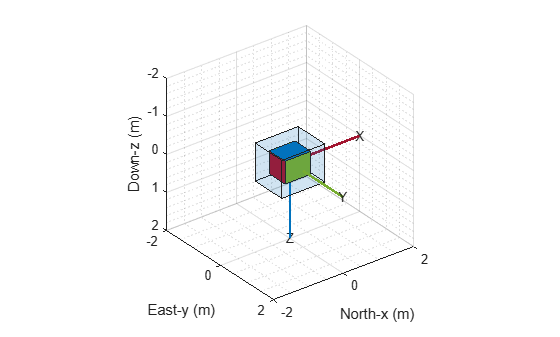Next, plot a pose with specified orientation and position.

q = quaternion([35 10 50],"eulerd","ZYX","frame");
position = [1 1 1];
poseplot(q,position)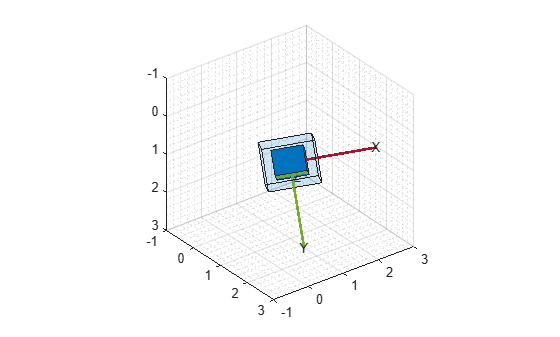Then, plot a second pose on the figure and return the PosePatch object. Plot the second pose with a smaller size by using the ScaleFactor name-value argument.

hold on
p = poseplot(eye(3),[5 5 5],ScaleFactor=0.5)
p =
PosePatch with properties:

Orientation: [3x3 double]
Position: [5 5 5]

Show all properties

legend("First Pose","Second Pose")
hold offAnimate a series of poses using the poseplot function. First, define the initial and final positions.

ps = [0 0 0];
pf = [10 0 0];

Then, define the initial and final orientations using the quaternion object.

qs = quaternion([45,0,0],'eulerd','ZYX','frame');
qf = quaternion([-45,0,0],'eulerd','ZYX','frame');

Show the starting pose.

patch = poseplot(qs,ps);
ylim([-2 2])
xlim([-2 12])
xlabel("North-x (m)")
ylabel("East-y (m)")
zlabel("Down-z (m)");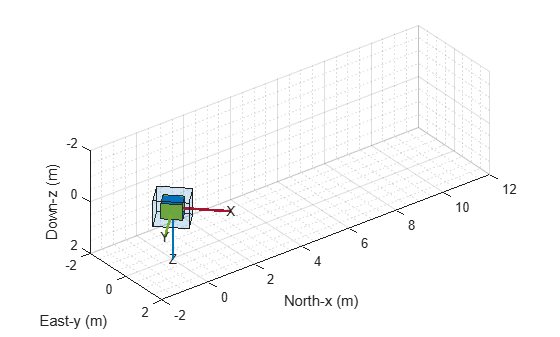Change the position and orientation continuously using coefficients, and update the pose using the set object function.

for coeff = 0:0.01:1
q = slerp(qs,qf,coeff);
position = ps + (pf - ps)*coeff;
set(patch,Orientation=q,Position=position);
drawnow
endPlot orientations and positions in meshes using the poseplot function. First, plot a ground vehicle at the origin with zero rotation.

poseplot(ones("quaternion"),[0 0 0],MeshFileName="groundvehicle.stl",ScaleFactor=0.3);
xlabel("North-x (m)")
ylabel("East-y (m)")
zlabel("Down-z (m)")Second, plot a rotor at the position [20 20 -20] with zero rotation.

hold on
poseplot(ones("quaternion"),[20 20 -20],MeshFileName="multirotor.stl",ScaleFactor=0.2);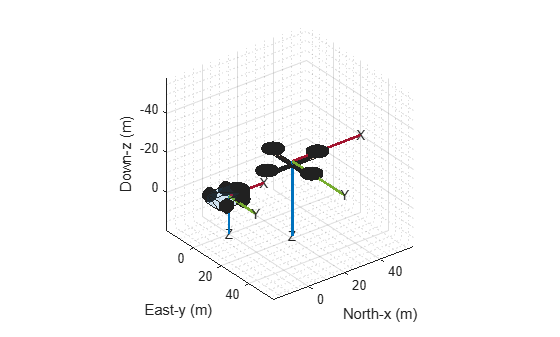Lastly, plot a fixed-wing aircraft at the position [5 5 -40] with zero rotation.

poseplot(ones("quaternion"),[5 5 -40],MeshFileName="fixedwing.stl",ScaleFactor=0.4);
view([-37.8 28.4])
hold off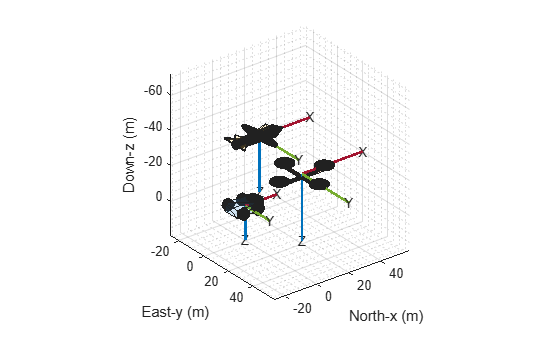Input Arguments

collapse all

Quaternion, specified as a quaternion object.

Rotation matrix, specified as a 3-by-3 orthonormal matrix.

Example: eye(3)

Position of the pose plot, specified as a three-element real-valued vector.

Example: [1 3 4]

Navigation frame of the pose plot, specified as "NED" for the north-east-down frame or "ENU" for the east-north-up frame.

When the parent axes status is hold off, specifying the NED navigation frame reverses the y- and z-axes in the figure by setting the YDir and ZDir properties of the parent axes.

Parent axes of the pose plot, specified as an Axes object. If you do not specify the axes, the poseplot function uses the current axes.

Name-Value Arguments

Specify optional pairs of arguments as Name1=Value1,...,NameN=ValueN, where Name is the argument name and Value is the corresponding value. Name-value arguments must appear after other arguments, but the order of the pairs does not matter.

Example: poseplot(PatchFaceAlpha=0.1)

The PosePatch properties listed here are only a subset. For a complete list, see PosePatch Properties.

Orientation of the pose plot, specified as a quaternion (Sensor Fusion and Tracking Toolbox) object or a rotation matrix.

Position of the pose plot, specified as a three-element real-valued vector.

Name of Standard Triangle Language (STL) mesh file, specified as a string scalar or a character vector containing the name of the mesh file. When you specify this argument, the poseplot function plots the mesh instead of the orientation box.

Scale factor of the pose plot, specified as a nonnegative scalar. The scale factor controls the size of the orientation box. When you specify the MeshFileName argument, the scale factor also changes the scale of the mesh.

Patch face color, specified as an RGB triplet, a hexadecimal color code, a color name, or a short name.

• An RGB triplet is a three-element row vector whose elements specify the intensities of the red, green, and blue components of the color. The intensities must be in the range [0, 1]; for example, [0.4 0.6 0.7].

• A hexadecimal color code is a character vector or a string scalar that starts with a hash symbol (#) followed by three or six hexadecimal digits, which can range from 0 to F. The values are not case sensitive. Thus, the color codes "#FF8800", "#ff8800", "#F80", and "#f80" are equivalent.

Here is a list of commonly used colors and their corresponding values.

Color NameShort NameRGB TripletHexadecimal Color CodeAppearance
"red""r"[1 0 0]"#FF0000""green""g"[0 1 0]"#00FF00""blue""b"[0 0 1]"#0000FF""cyan" "c"[0 1 1]"#00FFFF""magenta""m"[1 0 1]"#FF00FF""yellow""y"[1 1 0]"#FFFF00""black""k"[0 0 0]"#000000""white""w"[1 1 1]"#FFFFFF"Patch face transparency, specified as a scalar in range [0, 1]. A value of 1 is fully opaque and 0 is completely transparent.

Output Arguments

collapse all

Pose patch object, returned as a PosePatch object. You can use the returned object to query and modify properties of the plotted pose. For a list of properties, see PosePatch Properties.